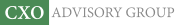Objective research to aid investing decisions

#### Value Investing Strategy (Strategy Overview)

Allocations for September 2023 (Final)
Cash TLT LQD SPY

#### Momentum Investing Strategy (Strategy Overview)

Allocations for September 2023 (Final)
1st ETF 2nd ETF 3rd ETF

# Combining Sharpe Ratio and Pairwise Correlation for Diversification

| | Posted in: Strategic Allocation

How can an investor decide whether a new strategy or new asset class (more generally, a stream of returns), is better than those currently in a portfolio? In their February 2012 paper entitled “The Sharpe Ratio Indifference Curve”, David Bailey and Marcos Lopez de Prado introduce a process for assessing addition of a new strategy (return stream) to an existing portfolio of strategies (return streams). They weight the return streams in the existing portfolio based on equal risk contribution (each return stream contributing equally to aggregate portfolio volatility). Their goal is to boost the Sharpe ratio of the portfolio by adding a new return stream. Using derivations and examples, they show that:

• The Sharpe ratio of the portfolio is a function of the number of component return streams, the average Sharpe ratio of these streams and the average pairwise correlation of these streams.
• The portfolio Sharpe ratio relates linearly to the average Sharpe ratio but non-linearly to the average pairwise correlation of the component return streams, such that:
• A new return stream with a low average pairwise correlation to the existing streams and below-average Sharpe ratio may boost the portfolio Sharpe ratio more than one with a high average pairwise correlation to the existing streams and above-average Sharpe ratio.
• Selecting new return streams solely based on past Sharpe ratio is unlikely to produce an optimally diversified portfolio.
• The indifference curve plots those combinations of a new return stream’s Sharpe ratio and its average correlation with existing portfolio streams that, if added, would not change the portfolio Sharpe ratio.

In summary, derivation shows that incremental diversification of a portfolio based on equal risk weighting depends on both the Sharpe ratio of the new return stream and its average pairwise correlation with return streams already in the portfolio.

Return streams as used above may derive from complex strategies, simple strategies or just assets.

Cautions regarding findings include:

• While not inherently in-sample, the approach requires assuming either that past Sharpe ratios and correlations predict future ones, or that future Sharpe ratios and correlations are predictable.
• Conclusions do not precisely apply to return stream weighting schemes other than equal risk contribution.
• Sharpe ratio has limitations as an outcome metric (see “The Sharpe Ratio: Blunted by Noise?”).

Compare the above approach with the empirical analysis (for equal weighting) in “Asset Class Diversification Effectiveness Factors” and the associated “Simple Tests of VXX as Diversifier”, “Simple Tests of FRN as Diversifier”“Simple Tests of TIP as Diversifier”, “Simple Tests of HYG as Diversifier” and “Simple Tests of USO as Diversifier”.